Next: 9. Trigonometric Functions Up: 8. Integrable Functions Previous: 8.5 Change of Scale   Index

# 8.6 Integrals and Area

8.51   Theorem. Let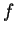be a piecewise monotonic function from an interval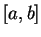to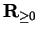. Then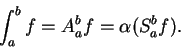Proof: We already know this result for monotonic functions, and from this the result follows easily for piecewise monotonic functions.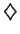Remark Theorem 8.51 is in fact true for all integrable functions fromto, but the proof is rather technical. Since we will never need the result for functions that are not piecewise monotonic, I will not bother to make an assumption out of it.

8.52   Theorem. Let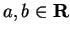and let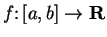be a piecewise monotonic function. Then the graph ofis a zero-area set.

Proof: We will show that the theorem holds whenis monotonic on. It then follows easily that the theorem holds whenis piecewise monotonic on.

Supposeis increasing on. Let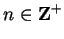and let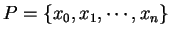be the regular partition ofinto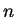equal subintervals.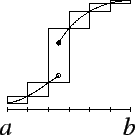Then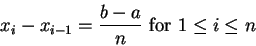and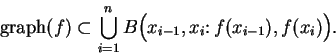Hence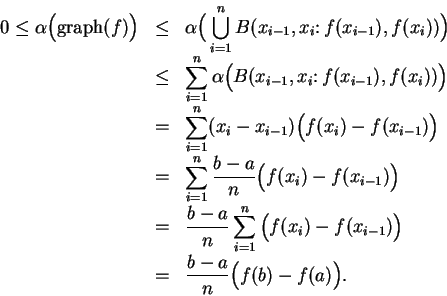Now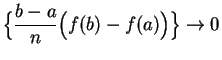, so it follows from the squeezing rule that the constant sequence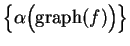converges to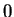, and hence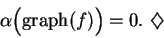Remark: Theorem 8.52 is actually valid for all integrable functions on.

8.53   Theorem (Area between graphs.) Let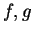be piecewise monotonic functions on an intervalsuch that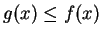for all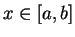. Let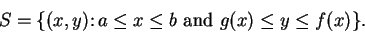Then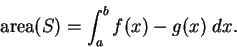Proof: Let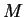be a lower bound for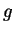, so that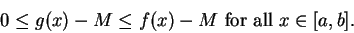Let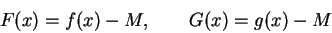for all, and let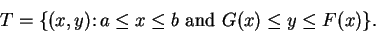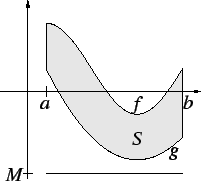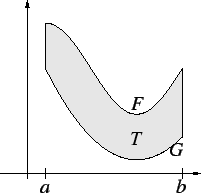Then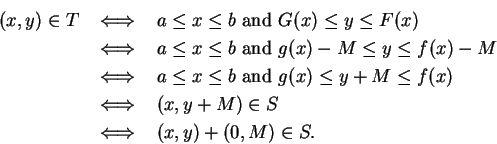It follows from translation invariance of area that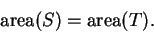Let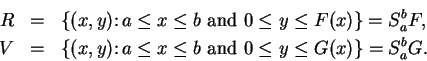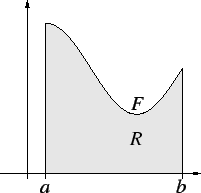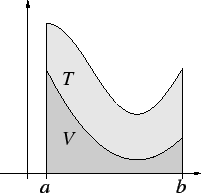Then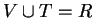, and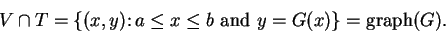It follows from theorem 8.52 that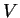and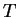are almost disjoint, so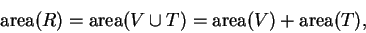and thus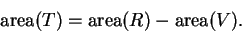By theorem 8.51 we have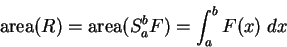and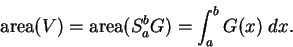Thus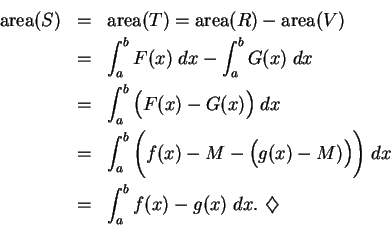Remark: Theorem 8.53 is valid for all integrable functionsand. This follows from our proof and the fact that theorems 8.51 and 8.52 are both valid for all integrable functions.

8.54   Example. We will find the area of the set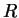in the figure, which is bounded by the graphs ofandwhere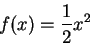and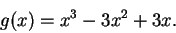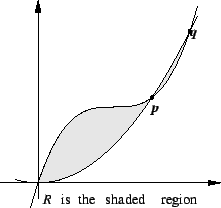Now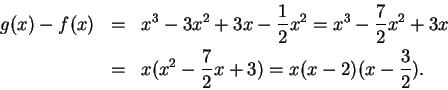Hence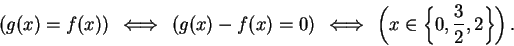It follows that the points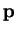and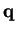in the figure are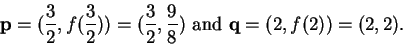Also, since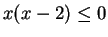for all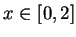,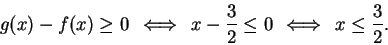(This is clear from the picture, assuming that the picture is accurate.) Thus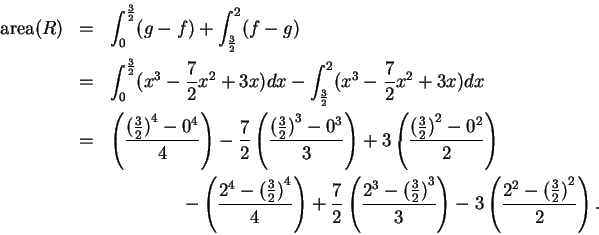We have now found the area, but the answer is not in a very informative form. It is not clear whether the number we have found is positive. It would be reasonable to use a calculator to simplify the result, but my experience with calculators is that I am more likely to make an error entering this into my calculator than I am to make an error by doing the calculation myself, so I will continue. I notice that three terms in the answer are repeated twice, so I have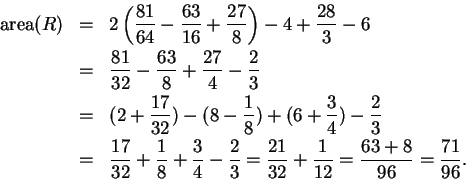Thus the area is about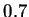From the sketch I expect the area to be a little bit smaller than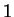, so the answer is plausible.

8.55   Exercise. The curve whose equation is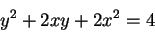(8.56)

is shown in the figure. Find the area enclosed by the curve.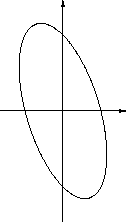(The set whose area we want to find is bounded by the graphs of the two functions. You can find the functions by considering equation (8.56) as a quadratic equation in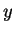and solving foras a function of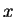.) A

8.57   Exercise. A Find the areas of the two sets shaded in the figures below: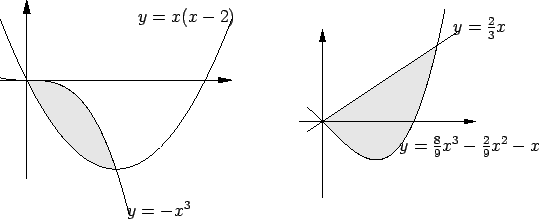8.58   Exercise. A Find the area of the shaded region.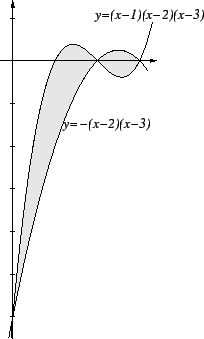Next: 9. Trigonometric Functions Up: 8. Integrable Functions Previous: 8.5 Change of Scale   Index
Ray Mayer 2007-09-07